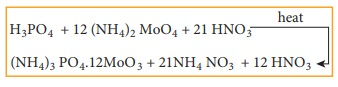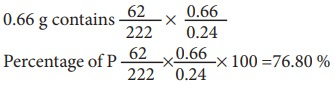Home | | Chemistry 11th std | Estimation of phosphorus

# Estimation of phosphorus

The precipitate of ammonium phosphomolybdate thus formed is filtered washed, dried and weighed.

Estimation of phosphorus:

## Carius method:

A known mass of the organic compound (w) containing phosphorous is heated with fuming HNO3 in a sealed tube where C is converted into CO2 and H to H2O. phosphorous present in organic compound is oxidized to phosphoric acid which is precipitated, as ammonium phosphomolybdate by heating with Conc. HNO3 and then adding ammonium molybdate.The precipitate of ammonium phosphomolybdate thus formed is filtered washed, dried and weighed.

In an alternative method, the phosphoric acid is precipitated as magnesium-ammonium phosphate by adding magnesia mixture (a mixture containing MgCl2, NH4Cl and ammonia) This ppt is washed, dried and ignited to get magnesium pyrophosphate which is washed, dried a weighed. The following are the reaction that takes place.

By knowing the mass of the organic compound and the mass of ammonium phosphomolybdate or magnesium pyrophosphate formed, the percentage of P is calculated.

Mass of organic compound is wg

Weight of ammonium

phosphomolybdate       =       x g

Weight of magnesium pyrophosphate = y g

Mole mass of (NH4)3PO4.12MoO3is =1877g

[3 x(14 + 4) + 31 +4(16)] + 12 (96+3x16)

Molar mass of Mg3P2O7 is 222 g

(2x24) + (31x2) + (7x16)

1877g of (NH4)3PO4.12MoO3contains 31g of P

Xg of(NH4)3PO4.12 MoO3 in w g of organic compound contains## Example 4:

0.24 g of organic compound containing phosphorous gave 0.66 g of Mg2P2O7 by the usual analysis. Calculate the percentage of phosphorous in the compound

Weight of an organic compound       = 0.24 g

Weight of Mg2P2O7 = 0.66 g

222 g of Mg2P2O7 contains 62 g of PTags : Equation, Procedure, Calculation, Example , 11th Chemistry : UNIT 11 : Fundamentals of Organic Chemistry
Study Material, Lecturing Notes, Assignment, Reference, Wiki description explanation, brief detail
11th Chemistry : UNIT 11 : Fundamentals of Organic Chemistry : Estimation of phosphorus | Equation, Procedure, Calculation, Example